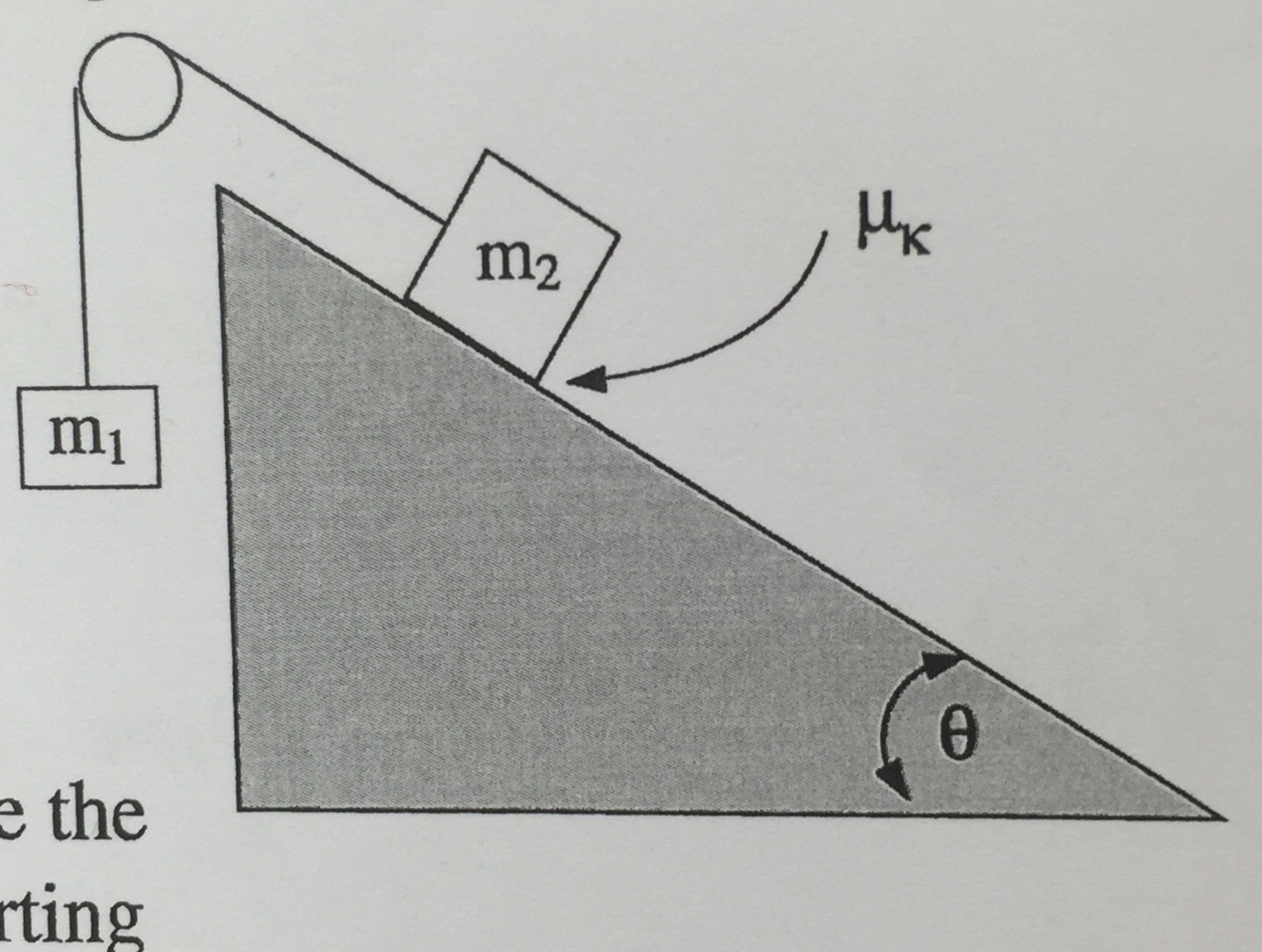# Problem: Two blocks of unequal mass are connected by a string over a smooth massless pulley with m2&gt;m1. The coefficient of kinetic friction between mass m2 and the incline is µk and the incline makes an angle theta with respect to the horizontal. (a) Evaluate the work done by gravity in moving the blocks a distance d. (b) Evaulate the work done by the frictional force in moving the distance d traveled. Is it positive or negative? (c) Using your answers from "a" and "b" evaluate the speed of the of the blocks after they move a distance d traveled. (d) Find an expression between masses m1 and m2 such that mass m2 slides down the incline at constant velocity

###### FREE Expert Solution

(a)

Gravitational potential energy:

$\overline{){\mathbf{U}}{\mathbf{=}}{\mathbf{m}}{\mathbf{g}}{\mathbf{h}}}$

Work done by gravity is equal to the change in gravitational potential energy.

98% (352 ratings)###### Problem Details

Two blocks of unequal mass are connected by a string over a smooth massless pulley with m2>m1. The coefficient of kinetic friction between mass m2 and the incline is µk and the incline makes an angle theta with respect to the horizontal.

(a) Evaluate the work done by gravity in moving the blocks a distance d.

(b) Evaulate the work done by the frictional force in moving the distance d traveled. Is it positive or negative?

(c) Using your answers from "a" and "b" evaluate the speed of the of the blocks after they move a distance d traveled.

(d) Find an expression between masses m1 and m2 such that mass m2 slides down the incline at constant velocity